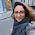## Wednesday, February 16, 2011

### Summarize Missing Data for all Variables in a Data Frame in R

Something like this probably already exists in an R package somewhere out there, but I needed a function to summarize how much missing data I have in each variable of a data frame in R. Pass a data frame to this function and for each variable it'll give you the number of missing values, the total N, and the proportion missing.

propmiss <- function(dataframe) lapply(dataframe,function(x) data.frame(nmiss=sum(is.na(x)), n=length(x), propmiss=sum(is.na(x))/length(x)))

Let's try it out.

#simulate some fake data
fakedata=data.frame(var1=c(1,2,NA,4,NA,6,7,8,9,10),var2=c(11,NA,NA,14,NA,16,17,NA,19,NA))

```print(fakedata)
var1 var2
1     1   11
2     2   NA
3    NA   NA
4     4   14
5    NA   NA
6     6   16
7     7   17
8     8   NA
9     9   19
10   10   NA
```

# summarize the missing data
propmiss(fakedata)
```\$var1
nmiss  n propmiss
1     2 10      0.2

\$var2
nmiss  n propmiss
1     5 10      0.5
```

Running that function returns a list of data.frame objects. You can access the proportion missing for var1 by running propmiss(fakedata)\$var1\$propmis.

*Edit 2011-02-23*

Commenter A. Friedman asked for a version of this function that gives you the output as a data frame. The function's a bit uglier because something was being coerced as a list, but this does the trick:

1.That's handy. Could you write an as.data.frame.propmiss() method that would coerce the output to a data.frame for easy use when there are a lot of variables being considered?

Thanks.

2.VERY handy thank you alot! this is why I love R and the R community.

3.A. Friedman - rewrote the function to do just that.

4.would you please comment the code? i need to do the same thing but calculating the sum of missings in every row instead.

5.returning output as a dataframe from original function is simple with dplyr: just wrap the call to propmiss into bind_rows.

x <- bind_rows(propmiss(df))

Note: Only a member of this blog may post a comment.